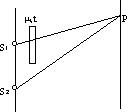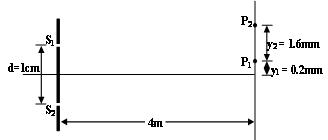×#### Thank you for registering.

One of our academic counsellors will contact you within 1 working day.

Click to Chat

1800-1023-196

+91-120-4616500

CART 0

• 0

MY CART (5)

Use Coupon: CART20 and get 20% off on all online Study Material

ITEM
DETAILS
MRP
DISCOUNT
FINAL PRICE
Total Price: Rs.

There are no items in this cart.
Continue Shopping• Complete Physics Course - Class 11
• OFFERED PRICE: Rs. 2,968
• View Details

```Displacement of Fringes

When a film of thickness’t’ and refractive index 'm' is introduced in the path of one of the sources, then fringe shift occurs as the optical path difference changes.Optical path difference at

P = S2P - [S1P+ µt - t] = S2P - S1P - (µ - 1)t = y.d/D - (µ - 1)

⇒  nth fringe is shifted by  Δy = D(µ-1)t/d = w/λ (µ-1)t

Illustration:

Monochromatic light of wavelength of 600 nm is used in a YDSE. One of the slits is covered by a transparent sheet of thickness 1.8 x 10-5 m made of a material of refractive index 1.6. How many fringes will shift due to the introduction of the sheet?

Solution:

As derived earlier, the total fringe shift = w/λ (µ-1)t .

As each fringe width = w,

The number of fringes that will shift = total fringe shift/fring width

(w/λ(µ-1)t)/w = (µ-1)t/λ = (1.6-1) x 1.8 x 10-5m / 600 x 10-9 = 18

Illustration:

In the YDSE conducted with white light (4000Å-7000Å), consider two points P1 and P2 on the screen at y1=0.2mm and y2=1.6mm, respectively. Determine the wavelengths which form maxima at these points.Solution:

The optical path difference at P1 is

p1 =dy1/d = ( 10/4000) (0.2) = 5 x 10-4mm = 5000Å

In the visible range 4000 - 7000Å

n1 = 5000/4000 = 1.25  and n2 = 5000/7000 = 0.714

The only integer between 0.714 and 1.25 is 1

∴ The wavelength which forms maxima at P is    l = 5000Å

For the point P2,

p2 = dy2/D = ( 10/4000) (1.6) = 4 x 10-3mm = 40000Å

Here n1 = 40000/4000 = 10 and n2 = 40000/7000 = 5.71

The integers between 5.71 and 10 are 6, 7, 8, 9 and 10

∴The wavelengths which form maxima at P2 are

λ1 = 4000 Å                for   n = 10

λ2 = 4444 Å                for   n = 9

λ3 = 5000 Å                for   n = 8

λ4 = 5714 Å                for   n = 7

λ5 = 6666 Å                for   n = 6
```### Course Features

• 101 Video Lectures
• Revision Notes
• Previous Year Papers
• Mind Map
• Study Planner
• NCERT Solutions
• Discussion Forum
• Test paper with Video Solution